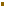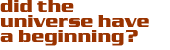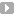### Instantons

Hawking and I made progress with the Hartle-Hawking proposal when we realised there is a simple class of classical solutions to the field equations for gravity coupled to an inflationary scalar field, which yield a finite probability according to the Dirac-Feynman formula in imaginary time.The solutions we studied yield a classical spacetime which is a real four dimensional manifold in either real time t or imaginary time τ provided that the transition t = -iτ is performed on the sero time surface Σ shown in Figure (3). The form of the solutions is generic for inflationary models with gently sloping potentials V(Φ), which are the simplest models.Figure 3: An infinite open Universe emerges from a ‘pea’ instanton.

Region I is an infinite inflating open ‘island Universe’. Region II an approximately de Sitter region Φ is nearly constant) bounded by a time-like singularity.

There are actually a one parameter family of finite probability solutions, in which we have a one-to-one relation(1)

where Ω0 is the current density parameter, and Φ0 is the value of the scalar field at the regular pole of the instanton. For the solutions described above, Ωo < 1, but if one allows instantons which are singular at both poles, and symmetric about the maximum of b, these analytically continue to closed inflating Universes, and one can obtain any value of Ωo > 1.

The classical instanton solutions could then be used to compute the quantum fluctuations about inflating spacetimes in a more precise manner than had hitherto been possible. In particular, previous calculations had to make more or less ad hoc choices for the initial quantum state. But in these new solutions the initial quantum state was initially and precisely defined from the no boundary proposal.I should also mention that doubts were raised about the Hawking-Turok solutions because they possess a singularity, albeit one mild enough that the quantum fluctuations about it are well defined.These doubts have since been dealt with by showing that by a suitable change of variables the singularity can actually be removed.These developments are reviewed in N. Turok, Before Inflation, preprint (2000). For the purposes of the present debate the precise predictions of the Hawking-Turok calculation are not relevant, though I must mention that work subsequent to this meeting has shown that one can actually calculate the volume factors appropriate to inflation in a slicing-independent way.When one includes these volume factors, the most probable instantons are those in which the initial field Φo starts out large, and the scalar field potential energy density is at the Planck density, at which quantum gravitational effects become large. Therefore these calculations do seem to resolve the question of the most probable starting point for inflation, which is at the Planck density.

Contributed by: Dr. Neil Turok

Cosmic QuestionsInflation and the Beginning of the Universe

InstantonsIntroductionEvidence for InflationEternal InflationThe No Boundary ProposalEternal Inflation Isn't

#### Source:Neil Turok